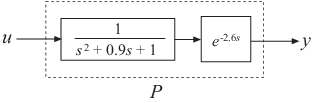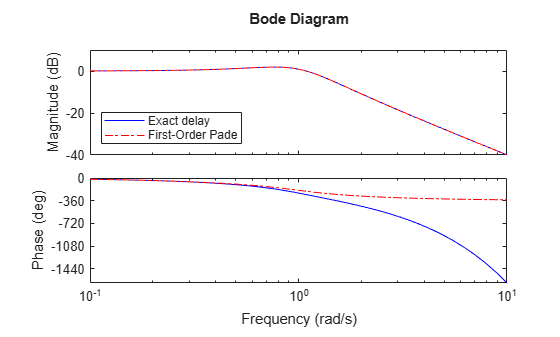## Time-Delay Approximation in Continuous-Time Open-Loop Model

This example shows how to approximate delays in a continuous-time open-loop system using `pade`.

Padé approximation is helpful when using analysis or design tools that do not support time delays.

1. Create sample open-loop system with an output delay.```s = tf('s'); P = exp(-2.6*s)/(s^2+0.9*s+1);```

`P` is a second-order transfer function (`tf`) object with a time delay.

2. Compute the first-order Padé approximation of `P`.

`Pnd1 = pade(P,1)`
```Pnd1 = -s + 0.7692 ---------------------------------- s^3 + 1.669 s^2 + 1.692 s + 0.7692 Continuous-time transfer function. ```

This command replaces all time delays in `P` with a first-order approximation. Therefore, `Pnd1` is a third-order transfer function with no delays.

3. Compare the frequency response of the original and approximate models using `bodeplot`.

```h = bodeoptions; h.PhaseMatching = 'on'; bodeplot(P,'-b',Pnd1,'-.r',{0.1,10},h) legend('Exact delay','First-Order Pade','Location','SouthWest')```The magnitude of `P` and `Pnd1` match exactly. However, the phase of `Pnd1` deviates from the phase of `P` beyond approximately 1 rad/s.

4. Increase the Padé approximation order to extend the frequency band in which the phase approximation is good.

`Pnd3 = pade(P,3);`
5. Compare the frequency response of `P`, `Pnd1` and `Pnd3`.

```bodeplot(P,'-b',Pnd3,'-.r',Pnd1,':k',{0.1 10},h) legend('Exact delay','Third-Order Pade','First-Order Pade',... 'Location','SouthWest')```The phase approximation error is reduced by using a third-order Padé approximation.

6. Compare the time domain responses of the original and approximated systems using `stepplot`.

```stepplot(P,'-b',Pnd3,'-.r',Pnd1,':k') legend('Exact delay','Third-Order Pade','First-Order Pade',... 'Location','Southeast')```Using the Padé approximation introduces a nonminimum phase artifact (“wrong way” effect) in the initial transient response. The effect is quite pronounced in the first-order approximation, which dips significantly below zero before changing direction. The effect is reduced in the higher-order approximation, which far more closely matches the exact system’s response.

Note

Using too high an approximation order may result in numerical issues and possibly unstable poles. Therefore, avoid Padé approximations with order N>10.